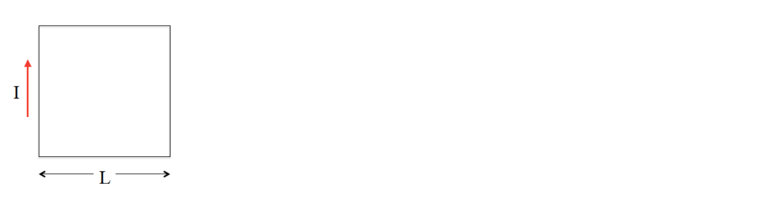# Problem: A conductor in the shape of a square loop of edge length L carries a current I as shown in the figure below. Calculate the magnitude and direction of the magnetic field at the center of the square.

🤓 Based on our data, we think this question is relevant for Professor Moussa's class at UCF.

###### Problem Details

A conductor in the shape of a square loop of edge length L carries a current I as shown in the figure below. Calculate the magnitude and direction of the magnetic field at the center of the square.